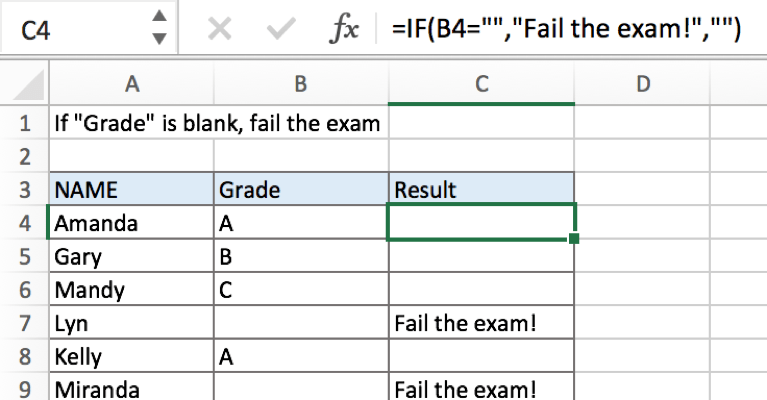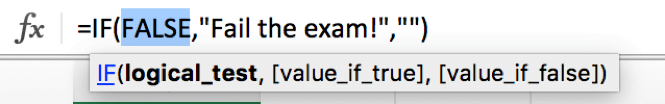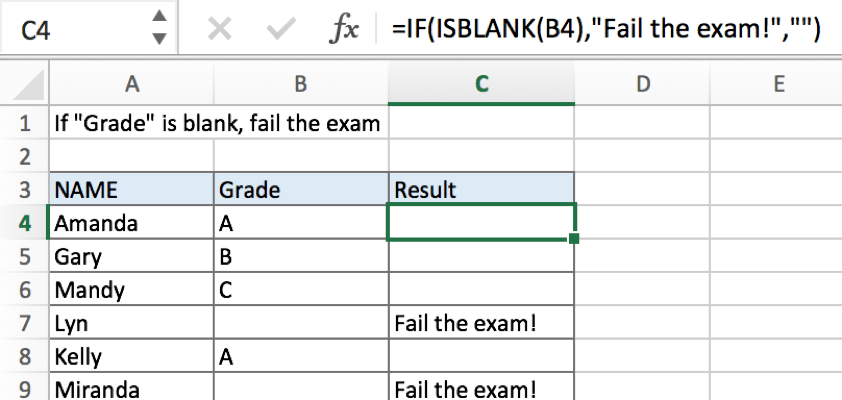# If Cell is Blank

IF function is frequently used in Excel worksheet to return you expect “true value” or “false value” based on the result of created logical test. If you want to see if a cell is blank or not, and leave some comments for blank cells, you can build a formula with IF function, IF function can return “value if blank” or “value if not blank” based on test “if cell is blank”.## FORMULA

To test if cell equals a certain text string, the generic formula is:

=IF(A1=””,”blank value”,”not blank value”)

Formula in this example:

=IF(B4=””,”Fail the exam!”,””)

## EXPLANATION

In this example, for a student, if cell in “Grade” column is blank, that means he/she failed the exam, and we want to add comment “Fail the exam!” in C column for those students who fail the exam. To return proper value based on “if cell is blank or not”, IF function can handle this case effectively.

IF function allows you to create a logical comparison between your value and reference value (for example “A1>0”), and set true value and false value what you expect to return as test results. IF function returns one of the two results based on logical comparison result.

Syntax: IF(logica_test,[value_if_true],[value_if_false])

To test if B4 is blank, we can directly create a logical comparison B4=“”, “” represents “Empty”, there is no need to add an extra space between “”, but double quotes “” should not be ignored. If missing double quotes, Excel will prompts warning message that the formula you typed contains an error, if you ignore the error, #VALUE! is displayed in cell.

In this example, B4 is not a blank cell, logical test B4=”” returns false to IF functon.As the result is false, IF function retrieves the value from “value if false” argument, in this case it is empty string, so nothing displays in C5. But for B7, B7 is a blank cell, it meets the condition “B7=”””, so IF retrieves true value “Fail the exam!” and return it to C7.

In IF, true value and false value can be set as empty string “”, a text, a number or a formula, you can set what you expect to these two values.

## ISBLANK Function

You can also use ISBLANK function to test if a cell is blank or not. In the formula, we can use ISBLANK(B4) to replace original logical test “B4=”””.### Related Functions

• Excel ISBLANK function
The Excel ISBLANK function returns TRUE if the value is blank or null.The syntax of the ISBLANK function is as below:= ISBLANK (value)…
• Excel IF function
The Excel IF function perform a logical test to return one value if the condition is TRUE and return another value if the condition is FALSE. The IF function is a build-in function in Microsoft Excel and it is categorized as a Logical Function.The syntax of the IF function is as below:= IF (condition, [true_value], [false_value])….